Next: Helium Atom Up: Identical Particles Previous: Spin Statistics Theorem

# Two-Electron System

Consider a system consisting of two electrons. Let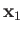andrepresent the position and spin operators of the first electron, respectively, and let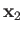and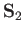represent the corresponding operators of the second electron. Furthermore, let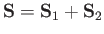represent the total spin operator of the system. Suppose that the Hamiltonian commutes with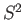, as is often the case. It follows that the state of the system is specified by the position eigenvalues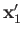and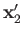, as well as the total spin quantum numbers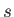and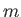. As usual, the eigenvalue ofis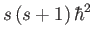, and the eigenvalue of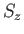is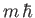. (See Chapter 5.) Moreover,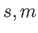can only take the values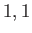or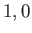or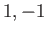or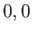, respectively. (See Chapter 6.) The overall wavefunction of the system can be written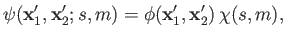(9.44)

where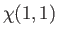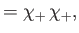(9.45)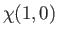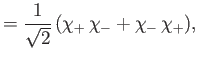(9.46)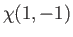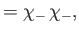(9.47)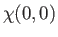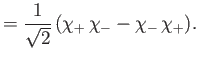(9.48)

(See Section 6.4.) Here, the spinor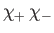denotes a state in which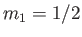and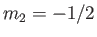, et cetera, where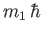and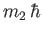are the eigenvalues of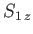and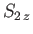, respectively. The three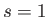spinors are usually referred to as triplet spinors, whereas the single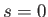spinor is called the singlet spinor. Note that the triplet spinors are all symmetric with respect to exchange of particles, whereas the singlet spinor is antisymmetric.

Fermi-Dirac statistics requires that the overall wavefunction be antisymmetric with respect to exchange of particles. Now, according to Equation (9.44), the overall wavefunction can be written as a product of a spatial wavefunction and a spinor. Moreover, when the system is in the spin-triplet state (i.e.,), the spinor is symmetric with respect to exchange of particles. On the other hand, when the system is in the spin-singlet state (i.e.,), the spinor is antisymmetric. It follows that, to maintain the overall antisymmetry of the wavefunction, the triplet spatial wavefunction must be antisymmetric with respect to exchange of particles, whereas the singlet spatial wavefunction must be symmetric. In other words, in the spin-triplet state the spatial wavefunction takes the form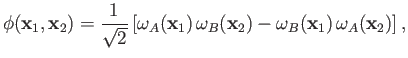(9.49)

whereas in the spin-singlet state the spatial wavefunction is written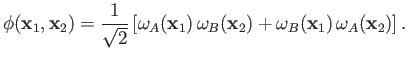(9.50)

The probability of observing one electron in the volume element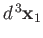around position, and the other in the volume element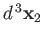around position, is proportional to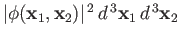, or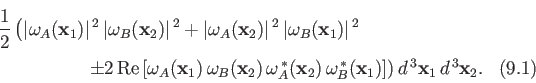Here, the plus sign corresponds to the spin-singlet state, whereas the minus sign corresponds to the spin-triplet state. We can immediately see that, in the spin-triplet state, the probability of finding the two electrons at the same point in space is zero. In other words, the two electrons have a tendency to avoid one another in this state. On the other hand, in the spin-singlet state, there is an enhanced probability of finding the two electrons at the same point in space (because of the final term in the previous expression). In other words, the two electrons are attracted to one another in this state. Note, however, that the spatial probability distributions associated with the singlet and triplet states differ substantially only when the two single-particle spatial wavefunctions,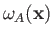and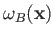, overlap: that is, when there exists a region of space in which the two wavefunctions are simultaneously non-negligible.Next: Helium Atom Up: Identical Particles Previous: Spin Statistics Theorem
Richard Fitzpatrick 2016-01-22